# Standard Order

## Smallest

6.2 × 10-4 = 0.00062

6.2 × 10-2 = 0.062

6.6 × 10-2 = 0.066

6.66 × 10-4 = 0.000666

6.6 × 10-3 = 0.0066

6.06 × 10-2 = 0.0606

6.06 × 10-3 = 0.00606

6.2 × 10-3 = 0.0062

6.26 × 10-2 = 0.0626

6.62 × 10-4 = 0.000662

## Largest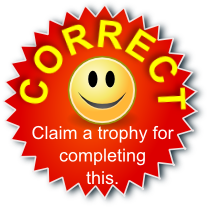There are more activities on the more general topic of Indices and some particularly useful links can be found below:

#### Standard Form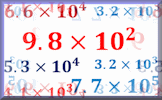A self marking exercise on converting standard form numbers to ordinary numbers and vica-versa.

Transum.org/go/?to=standardform

#### Indices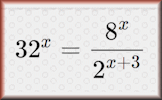A self marking exercise on indices (powers) including evaluating statements and solving equations.

Transum.org/go/?to=powers

#### Indices Pairs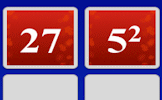The traditional pairs or pelmanism game adapted to test knowledge of indices. Enjoy the game!

Transum.org/go/?to=indices

The solutions to this and other Transum puzzles, exercises and activities are available here when you are signed in to your Transum subscription account. If you do not yet have an account and you are a teacher, tutor or parent you can apply for one here.

Do you have any comments? It is always useful to receive feedback and helps make this free resource even more useful for those learning Mathematics anywhere in the world. Click here to enter your comments.

## Description of Levels

CloseLevel 1 - Five numbers to sort, all with positive powers of 10

Level 2 - Ten numbers to sort, all with positive powers of 10

Level 3 - Ten numbers to sort, all with negative powers of 10

Level 4 - Ten mixed numbers to sort, all greater than 1

Level 5 - Ten mixed numbers to sort, all less than 1

Standard Form Exercise - A self marking exercise on converting standard form numbers to ordinary numbers and vica-versa

Standard Form Algebra - Deeply test your understanding of standard form by involving a little algebra.

Exam Style questions are in the style of GCSE or IB/A-level exam paper questions (worked solutions are available for Transum subscribers).

Answers to this exercise are available lower down this page when you are logged in to your Transum account. If you don’t yet have a Transum subscription one can be very quickly set up if you are a teacher, tutor or parent.

## Curriculum Reference

See the National Curriculum page for links to related online activities and resources.

## Standard Form

Standard form, also known as scientific notation, is a way of conveniently writing numbers that accommodates values too large or small to be conveniently written in standard decimal notation.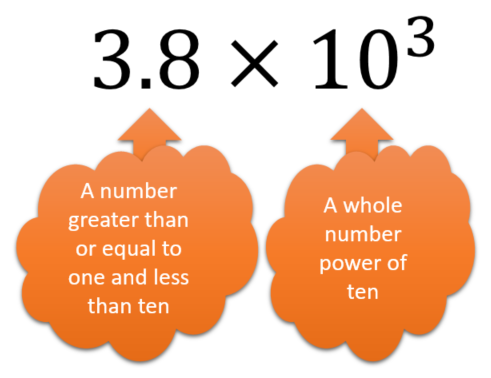## Examples

3.48 x 104

= 3.48 x 10 x 10 x 10 x 10

= 3.48 x 10000

= 34800

3.48 x 10-2

3.48 x 1100

= 3.48 ÷ 100

= 0.0348

Finally note that any number raised to the power zero is 1 so:

3.48 x 100

= 3.48 x 1

= 3.48

Answers to this exercise are available lower down this page when you are logged in to your Transum account. If you don’t yet have a Transum subscription one can be very quickly set up if you are a teacher, tutor or parent.

CloseFor All: